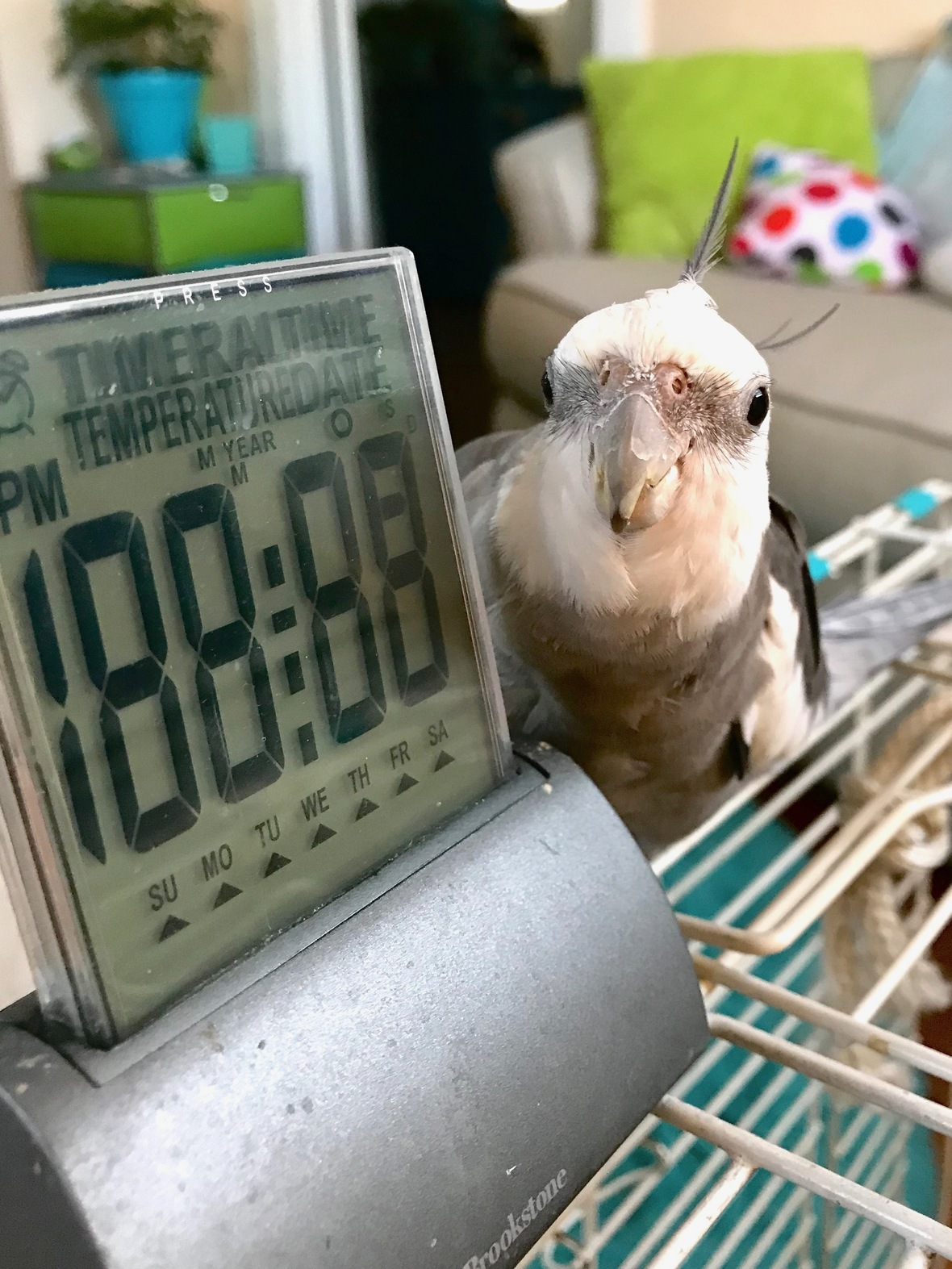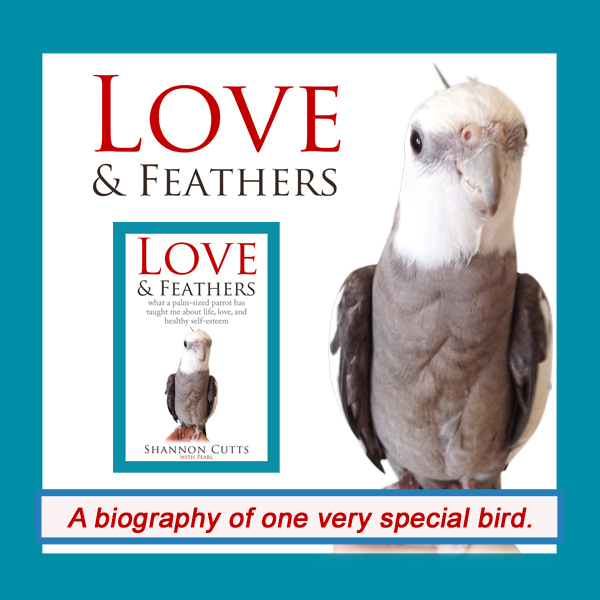# January 2019

 table div table+table+table div table{width:100%;padding:0}table div table+table+table div table img{width:96.23%;padding:0;float:none}table div table+table+table div table td{width:100%;padding:0 1.88% 18px}/* styles *//* styles */ Receiving this email as a forward? Sign up to keep following our little flock's adventures!
 table div table+table+table+table+table+table div table{width:100%;padding:0}table div table+table+table+table+table+table div table img{width:96.23%;padding:0;float:none}table div table+table+table+table+table+table div table td{width:100%;padding:0 1.88% 18px}/* styles */## Where Does Your Mind Go to Rest?Pearl posing on top of his small cage with his bestie, the bathroom clock.

I like to meditate in the morning. I have a specific reason for this preference.

For years I tried to do my meditations at night. Initially it sounded like such a good idea to meditate at night - to calm the mind before sleep. But with the pace of my daily life, by the time night typically arrives, my mind only wants to meditate on one thing....sleep.

This means I have gradually shifted my schedule so mornings are for meditation. This works great, save for one tiny remaining issue.

In the morning, after a whole night of relative inactivity, my mind is raring to go. It has so many things to think about! So many plans to cook up! So many worries to check in with! It has no time for meditation - there is too much thinking to do!

My mind and I usually start each morning meditation session with a combination of calming deep breathing and a rousing mental wrestling match. I say to my mind, "Okay, now breathe in deep." My body begins to breathe and my mind thinks, "Don't forget to add cilantro to your grocery list." I forget all about the breath, either cutting it short or holding it until my impatient body sends up an alarm signal.

So we try again. "Breathe in deep and then breathe out long." My body takes a full, deep breath, letting it out sharply as my mind reminds me, "You need to get up and take your thyroid pill - remember, you can't eat for an hour afterwards and you're already feeling hungry."

Sigh. And so it goes. The thing is, for so many years I assumed my mind really LIKES all this thinking! I figured it was actively sabotaging my efforts to meditate because it didn't ever want to turn off, unwind, take a load off.

I now know differently. It is true there are some thoughts my mind really likes to think and could think about forever without tiring of them. These thoughts generally focus on winning the lottery, coffee, wine, parrots, turtles, tiny houses, the beach.

But there are plenty of other thoughts it hates just as much as I do. It would like to stop thinking those thoughts just as much as I would like for it to stop thinking those thoughts. But it doesn't know HOW.

You see, thinking is what my mind does. It is all my mind knows how to do. Resting, silence, mental stillness, these don't feel native, natural, SAFE. Thinking feels safe. Thinking feels like busy, active, doing something, going somewhere, becoming someone. Thinking feels like living and surviving and not getting eaten for lunch by the smarter apex predator who didn't take any thinking breaks to relax and unwind.

So here we are. We both want to meditate. We both want to sink down deep beneath the surface layer of THOUGHTS and access a deeper intuition and wisdom. We both want - need - crave - peace. We both need to trust that underneath that bubbling top layer of restless thinking there is something more fundamental that includes body-mind-heart-spirit to nourish and restore us.

Eventually, usually at least, after many mental wrestling matches and a U-Haul truck's worth of mind-focusing meditation tactics, we do sink down into that wordless meditative depth together. When we get there, we both breathe out an ocean-sized sigh of relief. We made it. We didn't die. We are together. We may even find some answers or some new fascinating questions to bring back with us when we once again rise to the surface to continue our day.

In all this, I have learned my mind is not at all unlike my precious parrot, Pearl, who really hated his small car travel cage when I first introduced it to him. He would NOT. GO. IN. No way. That thing was scary! It was purple! It wasn't his cage!

Then one day I had the brilliant idea to pop in his bathroom clock, his favorite toy that he sings to and twitters to and hangs out with all day while I am busy writing. The moment I placed his bathroom clock into his small cage, guess who else went right in? Now whenever I bring out his small cage he gets so excited! Sometimes he even climbs in before I've had a chance to put his bathroom clock inside!

Today, when Pearl sees his small cage, he sees a friend, adventure, new sights and scenery and excitement and also safety. All that changed was that he doesn't go in there alone. He always brings a friend.

I am learning my mind is exactly the same way. It doesn't want to go anywhere alone. It gets scared so easily (it's all that thinking, I tell you).

I have to go in with it into the still inky darkness of the meditative space, coaxing it every step of the way to follow me in by using thought-rich breathing and meditative techniques as we go down, down, down, until at last we have arrived and my mind has just stopped thinking without even realizing it.

Then, we rest.

with great love and respect,

Shannon

 table div table+table+table+table+table+table+table+table+table div table{width:100%;padding:0}table div table+table+table+table+table+table+table+table+table div table img{width:96.23%;padding:0;float:none}table div table+table+table+table+table+table+table+table+table div table td{width:100%;padding:0 1.88% 18px}/* styles *//* styles */ Where does your mind go to rest? Do you have a specific strategy to help your mind unwind, de-stress, find ease and peace? If so, I sure would love to hear it! :-)
 table div table+table+table+table+table+table+table+table+table+table+table div table{width:100%;padding:0}table div table+table+table+table+table+table+table+table+table+table+table div table img{width:96.23%;padding:0;float:none}table div table+table+table+table+table+table+table+table+table+table+table div table td{width:100%;padding:0 1.88% 18px}/* styles */# "Waffles & More" - Order Your Tasty Copy!

 table.module-12{width:75.47%;padding:0}table div table+table+table+table+table+table+table+table+table+table+table+table+table div table{width:75.47%;float:none;margin-left:auto;margin-right:auto;padding:0}table div table+table+table+table+table+table+table+table+table+table+table+table+table div table a{border:0 none;text-decoration:none}table div table+table+table+table+table+table+table+table+table+table+table+table+table div table img{width:100%!important;border:0 none;text-decoration:none}table div table+table+table+table+table+table+table+table+table+table+table+table+table div table td{width:100%;padding:0}/* styles */
 table div table+table+table+table+table+table+table+table+table+table+table+table+table+table+table div table{width:100%;padding:0}table div table+table+table+table+table+table+table+table+table+table+table+table+table+table+table div table img{width:96.23%;padding:0;float:none}table div table+table+table+table+table+table+table+table+table+table+table+table+table+table+table div table td{width:100%;padding:0 1.88% 18px}/* styles */# Get your copy of Love & Feathers the book!Click on the image of Pearl to order your signed copy!Pearl will even beak-o-graph it for you!
 table div table+table+table+table+table+table+table+table+table+table+table+table+table+table+table+table+table+table+table+table div table{width:100%;padding:0}table div table+table+table+table+table+table+table+table+table+table+table+table+table+table+table+table+table+table+table+table div table img{width:96.23%;padding:0;float:none}table div table+table+table+table+table+table+table+table+table+table+table+table+table+table+table+table+table+table+table+table div table td{width:100%;padding:0 1.88% 18px}/* styles */# Malti has learned how to beg!

 table.module-21{width:75.47%;padding:0}table div table+table+table+table+table+table+table+table+table+table+table+table+table+table+table+table+table+table+table+table+table+table div table{width:75.47%;float:none;margin-left:auto;margin-right:auto;padding:0}table div table+table+table+table+table+table+table+table+table+table+table+table+table+table+table+table+table+table+table+table+table+table div table a{border:0 none;text-decoration:none}table div table+table+table+table+table+table+table+table+table+table+table+table+table+table+table+table+table+table+table+table+table+table div table img{width:100%!important;border:0 none;text-decoration:none}table div table+table+table+table+table+table+table+table+table+table+table+table+table+table+table+table+table+table+table+table+table+table div table td{width:100%;padding:0}/* styles */
 table div table+table+table+table+table+table+table+table+table+table+table+table+table+table+table+table+table+table+table+table+table+table+table+table div table{width:100%;padding:0}table div table+table+table+table+table+table+table+table+table+table+table+table+table+table+table+table+table+table+table+table+table+table+table+table div table img{width:96.23%;padding:0;float:none}table div table+table+table+table+table+table+table+table+table+table+table+table+table+table+table+table+table+table+table+table+table+table+table+table div table td{width:100%;padding:0 1.88% 18px}/* styles */# Bruce is in hibernation....we miss him!

 table.module-25{width:75.47%;padding:0}table div table+table+table+table+table+table+table+table+table+table+table+table+table+table+table+table+table+table+table+table+table+table+table+table+table+table div table{width:75.47%;float:none;margin-left:auto;margin-right:auto;padding:0}table div table+table+table+table+table+table+table+table+table+table+table+table+table+table+table+table+table+table+table+table+table+table+table+table+table+table div table a{border:0 none;text-decoration:none}table div table+table+table+table+table+table+table+table+table+table+table+table+table+table+table+table+table+table+table+table+table+table+table+table+table+table div table img{width:100%!important;border:0 none;text-decoration:none}table div table+table+table+table+table+table+table+table+table+table+table+table+table+table+table+table+table+table+table+table+table+table+table+table+table+table div table td{width:100%;padding:0}/* styles */
 table div table+table+table+table+table+table+table+table+table+table+table+table+table+table+table+table+table+table+table+table+table+table+table+table+table+table+table+table div table{width:100%;padding:0}table div table+table+table+table+table+table+table+table+table+table+table+table+table+table+table+table+table+table+table+table+table+table+table+table+table+table+table+table div table img{width:96.23%;padding:0;float:none}table div table+table+table+table+table+table+table+table+table+table+table+table+table+table+table+table+table+table+table+table+table+table+table+table+table+table+table+table div table td{width:100%;padding:0 1.88% 18px}/* styles */## Meet Pearl, Malti, Bruce & Me

 table div table+table+table+table+table+table+table+table+table+table+table+table+table+table+table+table+table+table+table+table+table+table+table+table+table+table+table+table+table+table div table,table.module-29{width:34.34%;float:left;padding:0}table div table+table+table+table+table+table+table+table+table+table+table+table+table+table+table+table+table+table+table+table+table+table+table+table+table+table+table+table+table+table div table a{border:0 none;text-decoration:none}table div table+table+table+table+table+table+table+table+table+table+table+table+table+table+table+table+table+table+table+table+table+table+table+table+table+table+table+table+table+table div table img{width:100%!important;border:0 none;text-decoration:none}table div table+table+table+table+table+table+table+table+table+table+table+table+table+table+table+table+table+table+table+table+table+table+table+table+table+table+table+table+table+table div table td{width:100%;padding:0 20px 20px 0}/* styles */ Hi! It is so good to meet you here each month! I'm so excited about our newest book, "Waffles & More: A Love & Feathers Recipe Book," which features so many of the recipes our flock (and especially the flock member with the feathers) particularly enjoys! And of course there is the book that started it all - "Love & Feathers: what a palm-sized parrot has taught me about life, love, and healthy self-esteem." This fun and feathery book focuses on the power of animal mentors in our lives and features my life with my precious parrot, Pearl. Malti is my darling young red-foot tortoise. She has such a healthy appetite for food, snoozing and life! Bruce, a very brave rescued Texas 3-toed box turtle, joined our flock a few years ago in a space clearly reserved just for him! My first book, "Beating Ana: how to outsmart your eating disorder and take your life back," is a practical hands-on workbook to help overcome eating disorders as well as the story of my personal recovery journey. MentorCONNECT, the first global eating disorders nonprofit mentoring community, emerged from the experience of writing "Beating Ana." While we closed in April 2017, the website continues to provide information about mentoring-based recovery support resources. ==> To connect with me: www.shannoncutts.com ==> To connect with Pearl, Malti & Bruce: www.loveandfeathersandshells.com
 table div table+table+table+table+table+table+table+table+table+table+table+table+table+table+table+table+table+table+table+table+table+table+table+table+table+table+table+table+table+table+table div table{width:100%;padding:0}table div table+table+table+table+table+table+table+table+table+table+table+table+table+table+table+table+table+table+table+table+table+table+table+table+table+table+table+table+table+table+table div table img{width:96.23%;padding:0;float:none}table div table+table+table+table+table+table+table+table+table+table+table+table+table+table+table+table+table+table+table+table+table+table+table+table+table+table+table+table+table+table+table div table td{width:100%;padding:0 1.88% 18px}/* styles */table div table+table+table+table+table+table+table+table+table+table+table+table+table+table+table+table+table+table+table+table+table+table+table+table+table+table+table+table+table+table+table+table div table td,table.module-31{width:100%;padding:0}table div table+table+table+table+table+table+table+table+table+table+table+table+table+table+table+table+table+table+table+table+table+table+table+table+table+table+table+table+table+table+table+table div table{width:100%;float:none;margin-left:auto;margin-right:auto;padding:0}table div table+table+table+table+table+table+table+table+table+table+table+table+table+table+table+table+table+table+table+table+table+table+table+table+table+table+table+table+table+table+table+table div table a{border:0 none;text-decoration:none}table div table+table+table+table+table+table+table+table+table+table+table+table+table+table+table+table+table+table+table+table+table+table+table+table+table+table+table+table+table+table+table+table div table img{width:100%!important;border:0 none;text-decoration:none}/* styles */
 table.module-32{width:74.15%;padding:0}table div table+table+table+table+table+table+table+table+table+table+table+table+table+table+table+table+table+table+table+table+table+table+table+table+table+table+table+table+table+table+table+table+table div table{width:74.15%;float:none;margin-left:auto;margin-right:auto;padding:0}table div table+table+table+table+table+table+table+table+table+table+table+table+table+table+table+table+table+table+table+table+table+table+table+table+table+table+table+table+table+table+table+table+table div table a{border:0 none;text-decoration:none}table div table+table+table+table+table+table+table+table+table+table+table+table+table+table+table+table+table+table+table+table+table+table+table+table+table+table+table+table+table+table+table+table+table div table img{width:100%!important;border:0 none;text-decoration:none}table div table+table+table+table+table+table+table+table+table+table+table+table+table+table+table+table+table+table+table+table+table+table+table+table+table+table+table+table+table+table+table+table+table div table td{width:100%;padding:0}/* styles */
 table div table+table+table+table+table+table+table+table+table+table+table+table+table+table+table+table+table+table+table+table+table+table+table+table+table+table+table+table+table+table+table+table+table+table div table{width:100%;padding:0}table div table+table+table+table+table+table+table+table+table+table+table+table+table+table+table+table+table+table+table+table+table+table+table+table+table+table+table+table+table+table+table+table+table+table div table img{width:96.23%;padding:0;float:none}table div table+table+table+table+table+table+table+table+table+table+table+table+table+table+table+table+table+table+table+table+table+table+table+table+table+table+table+table+table+table+table+table+table+table div table td{width:100%;padding:0 1.88% 18px}/* styles */## Reprints & Reposts

REPRINTS: Would you like to use any portion of this material in your newsletter, blog or website? You may do so as long as you also include this copyright information: